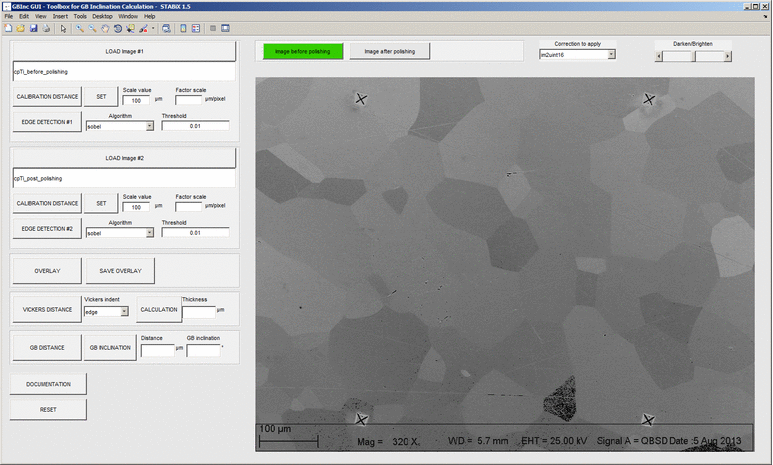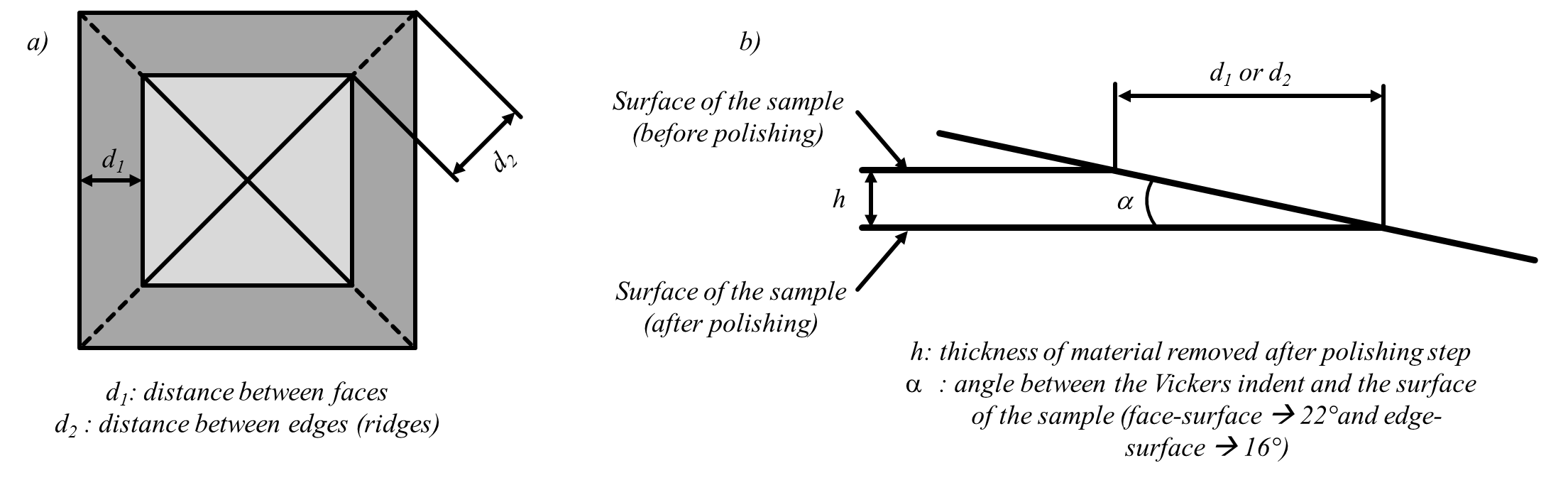# A Matlab toolbox to analyze grain boundary inclination from SEM images¶

Source code is hosted at Github.

This toolbox helps to find the grain boundary inclination from two micrographs from serial polishing. At least three marks such as microindents are needed for registration of the images.

Examples of micrographs from serial polishing.

To get started with this toolbox, clone the repository, then run Matlab, and cd into the folder containing this README file. Then add the package path to the Matlab search path by typing “path_management”. Finally you can start the launcher by typing demo or A_gui_gbinc at the Matlab command prompt.

## How to use the toolbox ?¶

1. Run the function A_gui_gbinc.m.
2. Select your first image before serial polishing.
3. Do the calibration to get the factor scale.
4. Do the edge detection.
5. Repeat the same operation for the second image obtained after serial polishing.
6. Do the overlay :
• If control points don’t exist (it’s the case for the 1st time), a window appears and it is possible to define control points.
• Define 3 control points per images.
• Select a point on the figure on the left, then on the figure on the right, and repeat this operation 2 times.
• Close the window for the selection of control points (Ctrl+W).
• Control points are saved in .mat file (in the same folder than the 1st picture loaded).
1. If the control points are not satisfying, delete them and redo the step 6 to set new control points and to get a new overlay.
2. Save the overlay in the same folder than the 1st picture loaded (as a screenshot.png) (optional).
3. Do the measurement of the distance between edges (Vickers faces) or ridges of a unique Vickers indent (see Figure 43).
4. Do the measurement of the distance between edges of a unique grain boundary.
5. The value of the grain boundary inclination is finally given in degrees.
• Calculation of the thickness of removed material after polishing

(29)$h = \frac{d}{\tan(90 - \alpha)}$

With $$d$$ the distance between edges (Vickers faces) or ridges of a unique Vickers indent (obtained before and after polishing), and $$\alpha$$ the angle between the Vickers indent and the surface of the sample (see Figure 43).

• Calculation of grain boundary inclination

(30)$GB_{inc} = \tan \left(\frac{d_{GB}}{h}\right)$

With $$d_{GB}$$ the distance between grain boundary traces (obtained before and after polishing), and $$h$$ the thickness of removed material after polishing calculatedFigure 41 Screenshots of the Matlab GUI used to calculate grain boundary inclination.Figure 43 Schemes of a) the top view of a Vickers indent (before and after polishing) and of b) the cross-section view.

Note

Images should have the same scale factor.

Note

Distances and grain boundary inclination values are obtained with the mean scale factor of the two images.

## Authors¶

Written by D. Mercier  and C. Zambaldi .

 Max-Planck-Institut für Eisenforschung, 40237 Düsseldorf, Germany

## Acknowledgements¶

Parts of this work were supported under the NSF/DFG Materials World Network program (DFG ZA 523/3-1 and NSF-DMR-1108211).

## Keywords¶

Matlab ; Graphical User Interface (GUI) ; Grain Boundaries ; Polycrystalline Metals ; Grain Boundary Inclination ; Serial Polishing ; Scanning Electron Microscope (SEM).